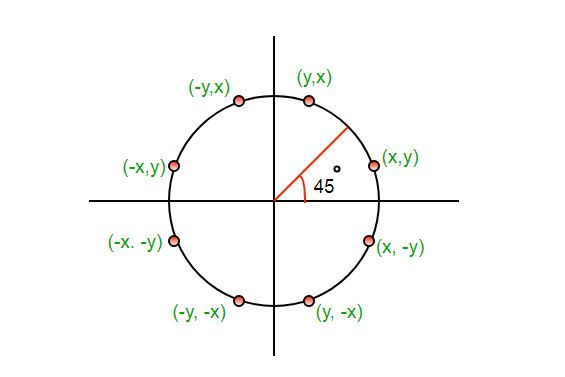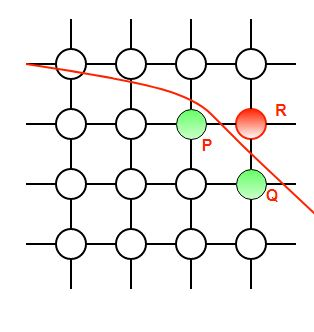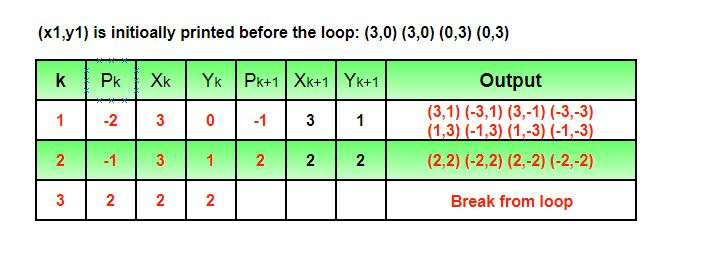GFG App
Open AppBrowser
Continue

# Mid-Point Circle Drawing Algorithm

The mid-point circle drawing algorithm is an algorithm used to determine the points needed for rasterizing a circle.

We use the mid-point algorithm to calculate all the perimeter points of the circle in the first octant and then print them along with their mirror points in the other octants. This will work because a circle is symmetric about its centre.The algorithm is very similar to the Mid-Point Line Generation Algorithm. Here, only the boundary condition is different.

For any given pixel (x, y), the next pixel to be plotted is either (x, y+1) or (x-1, y+1). This can be decided by following the steps below.

1. Find the mid-point p of the two possible pixels i.e (x-0.5, y+1)
2. If p lies inside or on the circle perimeter, we plot the pixel (x, y+1), otherwise if it’s outside we plot the pixel (x-1, y+1)

Boundary Condition : Whether the mid-point lies inside or outside the circle can be decided by using the formula:-

Given a circle centered at (0,0) and radius r and a point p(x,y)
F(p) = x2 + y2 – r2
if F(p)<0, the point is inside the circle
F(p)=0, the point is on the perimeter
F(p)>0, the point is outside the circleIn our program, we denote F(p) with P. The value of P is calculated at the mid-point of the two contending pixels i.e. (x-0.5, y+1). Each pixel is described with a subscript k.

Pk = (Xk — 0.5)2 + (yk + 1)2 – r2
Now,
xk+1 = xk or xk-1 , yk+1= yk +1
∴ Pk+1 = (xk+1 – 0.5)2 + (yk+1 +1)2 – r2
= (xk+1 – 0.5)2 + [(yk +1) + 1]2 – r2
= (xk+1 – 0.5)2 + (yk +1)2 + 2(yk + 1) + 1 – r2
= (xk+1 – 0.5)2 + [ – (xk – 0.5)2 +(xk – 0.5)2 ] + (yk + 1)2 – r2 + 2(yk + 1) + 1
= Pk + (xk+1 – 0.5)2 – (xk – 0.5)2 + 2(yk + 1) + 1
= Pk + (x2k+1 – x2k) – (xk+1 – xk) + 2(yk + 1) + 1
= Pk + 2(yk +1) + 1, when Pk <=0 i.e the midpoint is inside the circle
(xk+1 = xk
Pk + 2(yk +1) – 2(xk – 1) + 1, when Pk>0 I.e the mid point is outside the circle(xk+1 = xk-1)

The first point to be plotted is (r, 0) on the x-axis. The initial value of P is calculated as follows:-

P1 = (r – 0.5)2 + (0+1)2 – r2
= 1.25 – r
= 1 -r (When rounded off)

Examples:

```Input : Centre -> (0, 0), Radius -> 3
Output : (3, 0) (3, 0) (0, 3) (0, 3)
(3, 1) (-3, 1) (3, -1) (-3, -1)
(1, 3) (-1, 3) (1, -3) (-1, -3)
(2, 2) (-2, 2) (2, -2) (-2, -2)```` `
```Input : Centre -> (4, 4), Radius -> 2
Output : (6, 4) (6, 4) (4, 6) (4, 6)
(6, 5) (2, 5) (6, 3) (2, 3)
(5, 6) (3, 6) (5, 2) (3, 2)```

## CPP

 `// C++ program for implementing` `// Mid-Point Circle Drawing Algorithm` `#include` `using` `namespace` `std;`   `// Implementing Mid-Point Circle Drawing Algorithm` `void` `midPointCircleDraw(``int` `x_centre, ``int` `y_centre, ``int` `r)` `{` `    ``int` `x = r, y = 0;` `    `  `    ``// Printing the initial point on the axes ` `    ``// after translation` `    ``cout << ``"("` `<< x + x_centre << ``", "` `<< y + y_centre << ``") "``;` `    `  `    ``// When radius is zero only a single` `    ``// point will be printed` `    ``if` `(r > 0)` `    ``{` `        ``cout << ``"("` `<< x + x_centre << ``", "` `<< -y + y_centre << ``") "``;` `        ``cout << ``"("` `<< y + x_centre << ``", "` `<< x + y_centre << ``") "``;` `        ``cout << ``"("` `<< -y + x_centre << ``", "` `<< x + y_centre << ``")\n"``;` `    ``}` `    `  `    ``// Initialising the value of P` `    ``int` `P = 1 - r;` `    ``while` `(x > y)` `    ``{ ` `        ``y++;` `        `  `        ``// Mid-point is inside or on the perimeter` `        ``if` `(P <= 0)` `            ``P = P + 2*y + 1;` `        ``// Mid-point is outside the perimeter` `        ``else` `        ``{` `            ``x--;` `            ``P = P + 2*y - 2*x + 1;` `        ``}` `        `  `        ``// All the perimeter points have already been printed` `        ``if` `(x < y)` `            ``break``;` `        `  `        ``// Printing the generated point and its reflection` `        ``// in the other octants after translation` `        ``cout << ``"("` `<< x + x_centre << ``", "` `<< y + y_centre << ``") "``;` `        ``cout << ``"("` `<< -x + x_centre << ``", "` `<< y + y_centre << ``") "``;` `        ``cout << ``"("` `<< x + x_centre << ``", "` `<< -y + y_centre << ``") "``;` `        ``cout << ``"("` `<< -x + x_centre << ``", "` `<< -y + y_centre << ``")\n"``;` `        `  `        ``// If the generated point is on the line x = y then ` `        ``// the perimeter points have already been printed` `        ``if` `(x != y)` `        ``{` `            ``cout << ``"("` `<< y + x_centre << ``", "` `<< x + y_centre << ``") "``;` `            ``cout << ``"("` `<< -y + x_centre << ``", "` `<< x + y_centre << ``") "``;` `            ``cout << ``"("` `<< y + x_centre << ``", "` `<< -x + y_centre << ``") "``;` `            ``cout << ``"("` `<< -y + x_centre << ``", "` `<< -x + y_centre << ``")\n"``;` `        ``}` `    ``}` `}`   `// Driver code` `int` `main()` `{` `    ``// To draw a circle of radius 3 centered at (0, 0)` `    ``midPointCircleDraw(0, 0, 3);` `    ``return` `0;` `}`

## C

 `// C program for implementing` `// Mid-Point Circle Drawing Algorithm` `#include`   `// Implementing Mid-Point Circle Drawing Algorithm` `void` `midPointCircleDraw(``int` `x_centre, ``int` `y_centre, ``int` `r)` `{` `    ``int` `x = r, y = 0;` `    `  `    ``// Printing the initial point on the axes ` `    ``// after translation` `    ``printf``(``"(%d, %d) "``, x + x_centre, y + y_centre);` `    `  `    ``// When radius is zero only a single` `    ``// point will be printed` `    ``if` `(r > 0)` `    ``{` `        ``printf``(``"(%d, %d) "``, x + x_centre, -y + y_centre);` `        ``printf``(``"(%d, %d) "``, y + x_centre, x + y_centre);` `        ``printf``(``"(%d, %d)\n"``, -y + x_centre, x + y_centre);` `    ``}` `    `  `    ``// Initialising the value of P` `    ``int` `P = 1 - r;` `    ``while` `(x > y)` `    ``{ ` `        ``y++;` `        `  `        ``// Mid-point is inside or on the perimeter` `        ``if` `(P <= 0)` `            ``P = P + 2*y + 1;` `            `  `        ``// Mid-point is outside the perimeter` `        ``else` `        ``{` `            ``x--;` `            ``P = P + 2*y - 2*x + 1;` `        ``}` `        `  `        ``// All the perimeter points have already been printed` `        ``if` `(x < y)` `            ``break``;` `        `  `        ``// Printing the generated point and its reflection` `        ``// in the other octants after translation` `        ``printf``(``"(%d, %d) "``, x + x_centre, y + y_centre);` `        ``printf``(``"(%d, %d) "``, -x + x_centre, y + y_centre);` `        ``printf``(``"(%d, %d) "``, x + x_centre, -y + y_centre);` `        ``printf``(``"(%d, %d)\n"``, -x + x_centre, -y + y_centre);` `        `  `        ``// If the generated point is on the line x = y then ` `        ``// the perimeter points have already been printed` `        ``if` `(x != y)` `        ``{` `            ``printf``(``"(%d, %d) "``, y + x_centre, x + y_centre);` `            ``printf``(``"(%d, %d) "``, -y + x_centre, x + y_centre);` `            ``printf``(``"(%d, %d) "``, y + x_centre, -x + y_centre);` `            ``printf``(``"(%d, %d)\n"``, -y + x_centre, -x + y_centre);` `        ``}` `    ``} ` `}`   `// Driver code` `int` `main()` `{` `    ``// To draw a circle of radius 3 centered at (0, 0)` `    ``midPointCircleDraw(0, 0, 3);` `    ``return` `0;` `}`

## Java

 `// Java program for implementing` `// Mid-Point Circle Drawing Algorithm` `class` `GFG {` `    `  `    ``// Implementing Mid-Point Circle` `    ``// Drawing Algorithm` `    ``static` `void` `midPointCircleDraw(``int` `x_centre, ` `                            ``int` `y_centre, ``int` `r) ` `    ``{` `        `  `        ``int` `x = r, y = ``0``;` `    `  `        ``// Printing the initial point` `        ``// on the axes after translation` `        ``System.out.print(``"("` `+ (x + x_centre) ` `                ``+ ``", "` `+ (y + y_centre) + ``")"``);` `    `  `        ``// When radius is zero only a single` `        ``// point will be printed` `        ``if` `(r > ``0``) {` `            `  `            ``System.out.print(``"("` `+ (x + x_centre) ` `                ``+ ``", "` `+ (-y + y_centre) + ``")"``);` `                `  `            ``System.out.print(``"("` `+ (y + x_centre) ` `                 ``+ ``", "` `+ (x + y_centre) + ``")"``);` `                 `  `            ``System.out.println(``"("` `+ (-y + x_centre)` `                   ``+ ``", "` `+ (x + y_centre) + ``")"``);` `        ``}` `    `  `        ``// Initialising the value of P` `        ``int` `P = ``1` `- r;` `        ``while` `(x > y) {` `            `  `            ``y++;` `        `  `            ``// Mid-point is inside or on the perimeter` `            ``if` `(P <= ``0``)` `                ``P = P + ``2` `* y + ``1``;` `        `  `            ``// Mid-point is outside the perimeter` `            ``else` `{` `                ``x--;` `                ``P = P + ``2` `* y - ``2` `* x + ``1``;` `            ``}` `        `  `            ``// All the perimeter points have already ` `            ``// been printed` `            ``if` `(x < y)` `                ``break``;` `        `  `            ``// Printing the generated point and its ` `            ``// reflection in the other octants after` `            ``// translation` `            ``System.out.print(``"("` `+ (x + x_centre) ` `                    ``+ ``", "` `+ (y + y_centre) + ``")"``);` `                    `  `            ``System.out.print(``"("` `+ (-x + x_centre) ` `                    ``+ ``", "` `+ (y + y_centre) + ``")"``);` `                    `  `            ``System.out.print(``"("` `+ (x + x_centre) + ` `                    ``", "` `+ (-y + y_centre) + ``")"``);` `                    `  `            ``System.out.println(``"("` `+ (-x + x_centre) ` `                    ``+ ``", "` `+ (-y + y_centre) + ``")"``);` `        `  `            ``// If the generated point is on the ` `            ``// line x = y then the perimeter points` `            ``// have already been printed` `            ``if` `(x != y) {` `                `  `                ``System.out.print(``"("` `+ (y + x_centre)` `                      ``+ ``", "` `+ (x + y_centre) + ``")"``);` `                      `  `                ``System.out.print(``"("` `+ (-y + x_centre) ` `                      ``+ ``", "` `+ (x + y_centre) + ``")"``);` `                      `  `                ``System.out.print(``"("` `+ (y + x_centre) ` `                      ``+ ``", "` `+ (-x + y_centre) + ``")"``);` `                      `  `                ``System.out.println(``"("` `+ (-y + x_centre) ` `                    ``+ ``", "` `+ (-x + y_centre) +``")"``);` `            ``}` `        ``}` `    ``}` `    `  `    ``// Driver code` `    ``public` `static` `void` `main(String[] args) {` `        `  `        ``// To draw a circle of radius ` `        ``// 3 centered at (0, 0)` `        ``midPointCircleDraw(``0``, ``0``, ``3``);` `    ``}` `}`   `// This code is contributed by Anant Agarwal.`

## Python3

 `# Python3 program for implementing ` `# Mid-Point Circle Drawing Algorithm `   `def` `midPointCircleDraw(x_centre, y_centre, r):` `    ``x ``=` `r` `    ``y ``=` `0` `    `  `    ``# Printing the initial point the ` `    ``# axes after translation ` `    ``print``(``"("``, x ``+` `x_centre, ``", "``, ` `               ``y ``+` `y_centre, ``")"``, ` `               ``sep ``=` `"``", end = "``") ` `    `  `    ``# When radius is zero only a single ` `    ``# point be printed ` `    ``if` `(r > ``0``) :` `    `  `        ``print``(``"("``, x ``+` `x_centre, ``", "``,` `                  ``-``y ``+` `y_centre, ``")"``, ` `                  ``sep ``=` `"``", end = "``") ` `        ``print``(``"("``, y ``+` `x_centre, ``", "``, ` `                   ``x ``+` `y_centre, ``")"``,` `                   ``sep ``=` `"``", end = "``") ` `        ``print``(``"("``, ``-``y ``+` `x_centre, ``", "``, ` `                    ``x ``+` `y_centre, ``")"``, sep ``=` `"") ` `    `  `    ``# Initialising the value of P ` `    ``P ``=` `1` `-` `r `   `    ``while` `x > y:` `    `  `        ``y ``+``=` `1` `        `  `        ``# Mid-point inside or on the perimeter` `        ``if` `P <``=` `0``: ` `            ``P ``=` `P ``+` `2` `*` `y ``+` `1` `            `  `        ``# Mid-point outside the perimeter ` `        ``else``:         ` `            ``x ``-``=` `1` `            ``P ``=` `P ``+` `2` `*` `y ``-` `2` `*` `x ``+` `1` `        `  `        ``# All the perimeter points have ` `        ``# already been printed ` `        ``if` `(x < y):` `            ``break` `        `  `        ``# Printing the generated point its reflection ` `        ``# in the other octants after translation ` `        ``print``(``"("``, x ``+` `x_centre, ``", "``, y ``+` `y_centre,` `                            ``")"``, sep ``=` `"``", end = "``") ` `        ``print``(``"("``, ``-``x ``+` `x_centre, ``", "``, y ``+` `y_centre, ` `                             ``")"``, sep ``=` `"``", end = "``") ` `        ``print``(``"("``, x ``+` `x_centre, ``", "``, ``-``y ``+` `y_centre,` `                             ``")"``, sep ``=` `"``", end = "``") ` `        ``print``(``"("``, ``-``x ``+` `x_centre, ``", "``, ``-``y ``+` `y_centre,` `                                        ``")"``, sep ``=` `"") ` `        `  `        ``# If the generated point on the line x = y then ` `        ``# the perimeter points have already been printed ` `        ``if` `x !``=` `y:` `        `  `            ``print``(``"("``, y ``+` `x_centre, ``", "``, x ``+` `y_centre, ` `                                ``")"``, sep ``=` `"``", end = "``") ` `            ``print``(``"("``, ``-``y ``+` `x_centre, ``", "``, x ``+` `y_centre,` `                                 ``")"``, sep ``=` `"``", end = "``") ` `            ``print``(``"("``, y ``+` `x_centre, ``", "``, ``-``x ``+` `y_centre,` `                                 ``")"``, sep ``=` `"``", end = "``") ` `            ``print``(``"("``, ``-``y ``+` `x_centre, ``", "``, ``-``x ``+` `y_centre, ` `                                            ``")"``, sep ``=` `"")` `                            `  `# Driver Code` `if` `__name__ ``=``=` `'__main__'``:` `    `  `    ``# To draw a circle of radius 3 ` `    ``# centered at (0, 0) ` `    ``midPointCircleDraw(``0``, ``0``, ``3``)`     `# Contributed by: SHUBHAMSINGH10` `# Improved by: siddharthx_07`

## C#

 `// C# program for implementing Mid-Point` `// Circle Drawing Algorithm` `using` `System;`   `class` `GFG {` `    `  `    ``// Implementing Mid-Point Circle` `    ``// Drawing Algorithm` `    ``static` `void` `midPointCircleDraw(``int` `x_centre, ` `                            ``int` `y_centre, ``int` `r) ` `    ``{` `        `  `        ``int` `x = r, y = 0;` `    `  `        ``// Printing the initial point on the` `        ``// axes after translation` `        ``Console.Write(``"("` `+ (x + x_centre) ` `                ``+ ``", "` `+ (y + y_centre) + ``")"``);` `    `  `        ``// When radius is zero only a single` `        ``// point will be printed` `        ``if` `(r > 0)` `        ``{` `            `  `            ``Console.Write(``"("` `+ (x + x_centre) ` `                ``+ ``", "` `+ (-y + y_centre) + ``")"``);` `                `  `            ``Console.Write(``"("` `+ (y + x_centre) ` `                ``+ ``", "` `+ (x + y_centre) + ``")"``);` `                `  `            ``Console.WriteLine(``"("` `+ (-y + x_centre)` `                ``+ ``", "` `+ (x + y_centre) + ``")"``);` `        ``}` `    `  `        ``// Initialising the value of P` `        ``int` `P = 1 - r;` `        ``while` `(x > y)` `        ``{` `            `  `            ``y++;` `        `  `            ``// Mid-point is inside or on the perimeter` `            ``if` `(P <= 0)` `                ``P = P + 2 * y + 1;` `        `  `            ``// Mid-point is outside the perimeter` `            ``else` `            ``{` `                ``x--;` `                ``P = P + 2 * y - 2 * x + 1;` `            ``}` `        `  `            ``// All the perimeter points have already ` `            ``// been printed` `            ``if` `(x < y)` `                ``break``;` `        `  `            ``// Printing the generated point and its ` `            ``// reflection in the other octants after` `            ``// translation` `            ``Console.Write(``"("` `+ (x + x_centre) ` `                    ``+ ``", "` `+ (y + y_centre) + ``")"``);` `                    `  `            ``Console.Write(``"("` `+ (-x + x_centre) ` `                    ``+ ``", "` `+ (y + y_centre) + ``")"``);` `                    `  `            ``Console.Write(``"("` `+ (x + x_centre) + ` `                    ``", "` `+ (-y + y_centre) + ``")"``);` `                    `  `            ``Console.WriteLine(``"("` `+ (-x + x_centre) ` `                    ``+ ``", "` `+ (-y + y_centre) + ``")"``);` `        `  `            ``// If the generated point is on the ` `            ``// line x = y then the perimeter points` `            ``// have already been printed` `            ``if` `(x != y) ` `            ``{` `                ``Console.Write(``"("` `+ (y + x_centre)` `                    ``+ ``", "` `+ (x + y_centre) + ``")"``);` `                        `  `                ``Console.Write(``"("` `+ (-y + x_centre) ` `                    ``+ ``", "` `+ (x + y_centre) + ``")"``);` `                        `  `                ``Console.Write(``"("` `+ (y + x_centre) ` `                    ``+ ``", "` `+ (-x + y_centre) + ``")"``);` `                        `  `                ``Console.WriteLine(``"("` `+ (-y + x_centre) ` `                    ``+ ``", "` `+ (-x + y_centre) +``")"``);` `            ``}` `        ``}` `    ``}` `    `  `    ``// Driver code` `    ``public` `static` `void` `Main()` `    ``{` `        `  `        ``// To draw a circle of radius ` `        ``// 3 centered at (0, 0)` `        ``midPointCircleDraw(0, 0, 3);` `    ``}` `}`   `// This code is contributed by nitin mittal.`

## PHP

 ` 0)` `    ``{` `        ``echo` `"("``,``\$x` `+ ``\$x_centre``,``","``, -``\$y` `+ ``\$y_centre``,``")"``;` `        ``echo` `"("``,``\$y` `+ ``\$x_centre``,``","``, ``\$x` `+ ``\$y_centre``,``")"``;` `        ``echo` `"("``,-``\$y` `+ ``\$x_centre``,``","``, ``\$x` `+ ``\$y_centre``,``")"``,``"\n"``;` `    ``}` `    `  `    ``// Initializing the value of P` `    ``\$P` `= 1 - ``\$r``;` `    ``while` `(``\$x` `> ``\$y``)` `    ``{ ` `        ``\$y``++;` `        `  `        ``// Mid-point is inside ` `        ``// or on the perimeter` `        ``if` `(``\$P` `<= 0)` `            ``\$P` `= ``\$P` `+ 2 * ``\$y` `+ 1;` `            `  `        ``// Mid-point is outside` `        ``// the perimeter` `        ``else` `        ``{` `            ``\$x``--;` `            ``\$P` `= ``\$P` `+ 2 * ``\$y` `- ` `                  ``2 * ``\$x` `+ 1;` `        ``}` `        `  `        ``// All the perimeter points` `        ``// have already been printed` `        ``if` `(``\$x` `< ``\$y``)` `            ``break``;` `        `  `        ``// Printing the generated ` `        ``// point and its reflection` `        ``// in the other octants ` `        ``// after translation` `        ``echo` `"("``,``\$x` `+ ``\$x_centre``,``","``, ``\$y` `+ ``\$y_centre``,``")"``;` `        ``echo` `"("``,-``\$x` `+ ``\$x_centre``,``","``, ``\$y` `+ ``\$y_centre``,``")"``;` `        ``echo` `"("``,``\$x` `+``\$x_centre``,``","``, -``\$y` `+ ``\$y_centre``,``")"``;` `        ``echo` `"("``,-``\$x` `+ ``\$x_centre``,``","``, -``\$y` `+ ``\$y_centre``,``")"``,``"\n"``;` `        `  `        ``// If the generated point is ` `        ``// on the line x = y then ` `        ``// the perimeter points have ` `        ``// already been printed` `        ``if` `(``\$x` `!= ``\$y``)` `        ``{` `            ``echo` `"("``,``\$y` `+ ``\$x_centre``,``","``, ``\$x` `+ ``\$y_centre``,``")"``;` `            ``echo` `"("``,-``\$y` `+ ``\$x_centre``,``","``, ``\$x` `+ ``\$y_centre``,``")"``;` `            ``echo` `"("``,``\$y` `+ ``\$x_centre``,``","``, -``\$x` `+ ``\$y_centre``,``")"``;` `            ``echo` `"("``,-``\$y` `+ ``\$x_centre``,``","``, -``\$x` `+ ``\$y_centre``,``")"``,``"\n"``;` `        ``}` `    ``} ` `}`   `    ``// Driver code` `    ``// To draw a circle of radius` `    ``// 3 centered at (0, 0)` `    ``midPointCircleDraw(0, 0, 3);` `    `  `// This code is contributed by nitin mittal.` `?>`

## Javascript

 ``

Output:

```(3, 0) (3, 0) (0, 3) (0, 3)
(3, 1) (-3, 1) (3, -1) (-3, -1)
(1, 3) (-1, 3) (1, -3) (-1, -3)
(2, 2) (-2, 2) (2, -2) (-2, -2)```

Time Complexity: O(x – y)
Auxiliary Space: O(1)
References : Midpoint Circle Algorithm
Image References : Octants of a circle, Rasterised Circle, the other images were created for this article by the geek